NCERT Exemplar - MCQs

Chapter 5 Class 12 Continuity and Differentiability
Serial order wise

## (D) continuous at x = 0 but not at x = 1.

This question is similar to Ex 5.1, 34 - Chapter 5 Class 12 - Continuity and Differentiability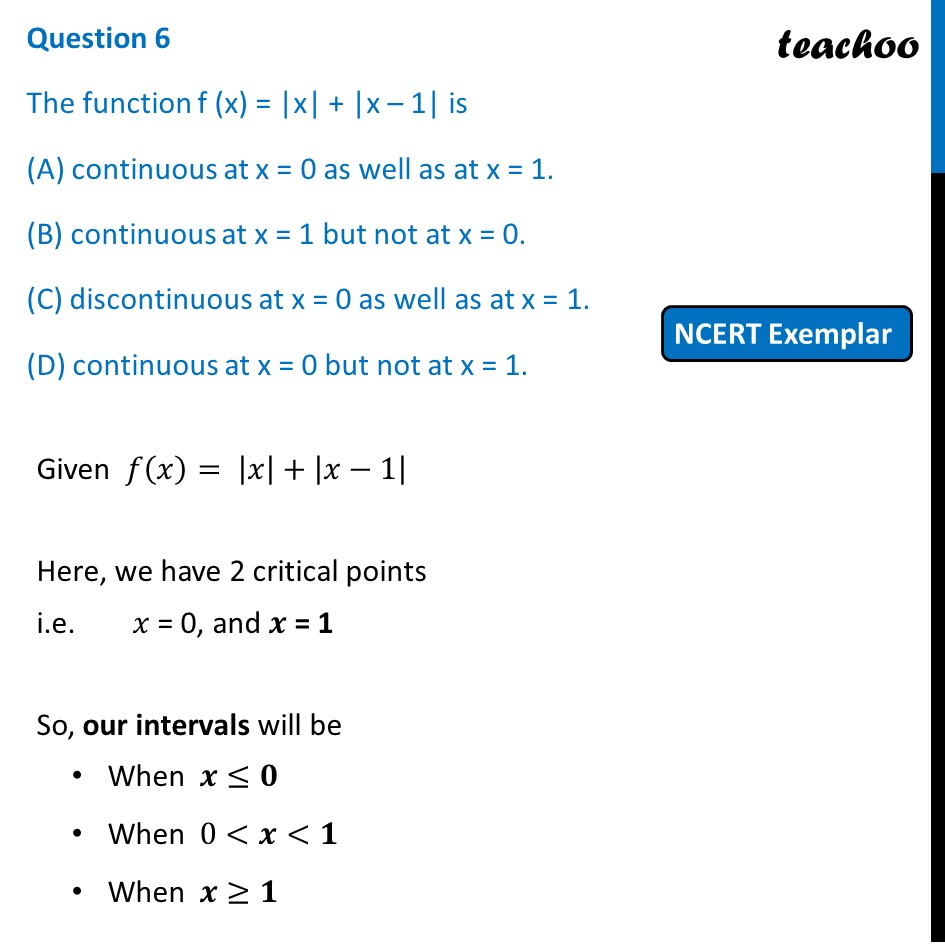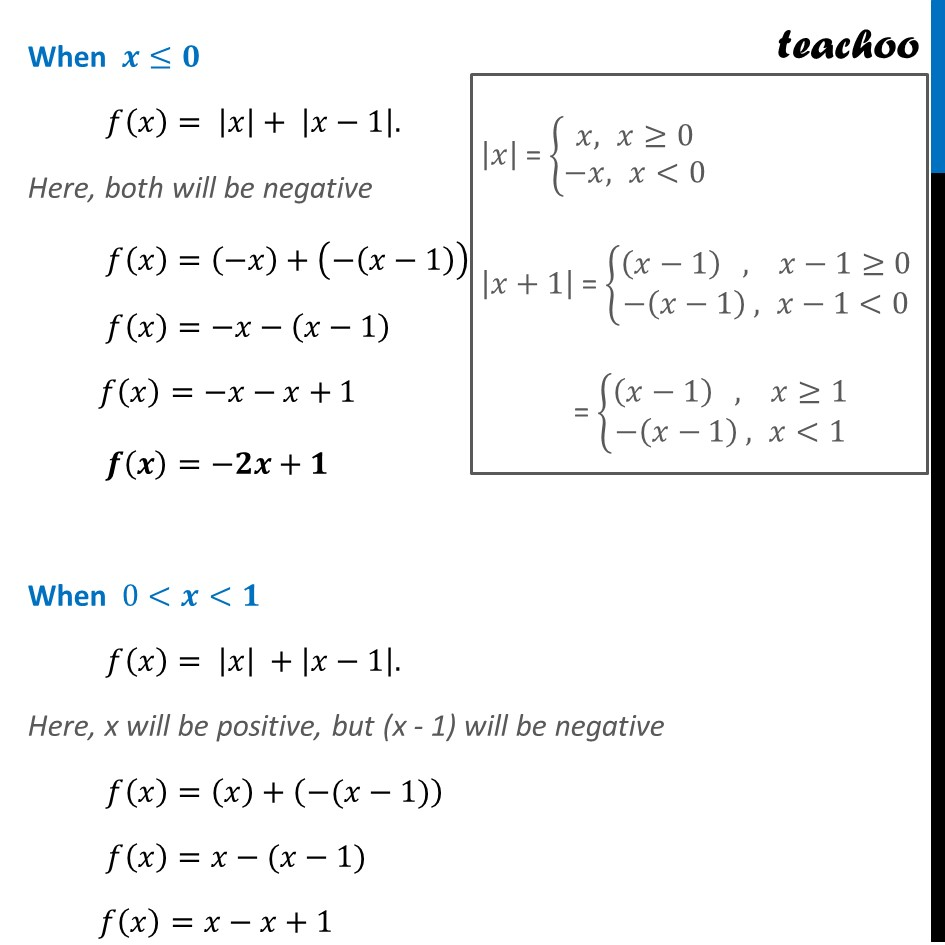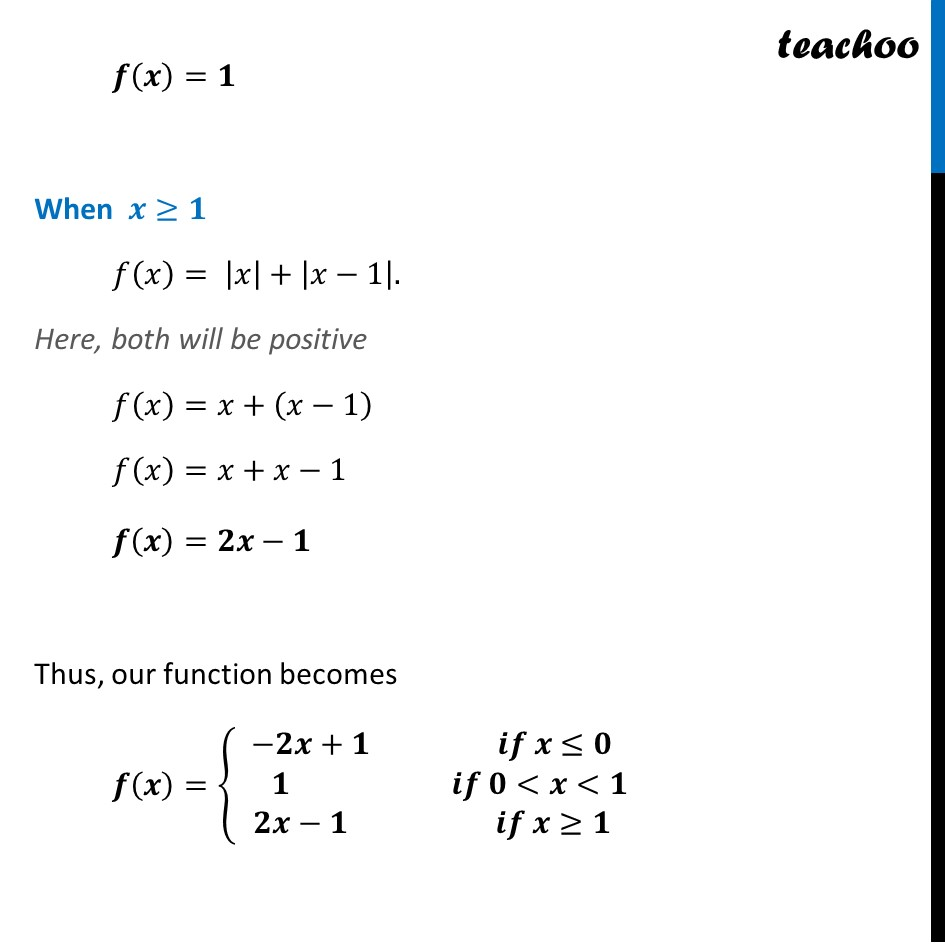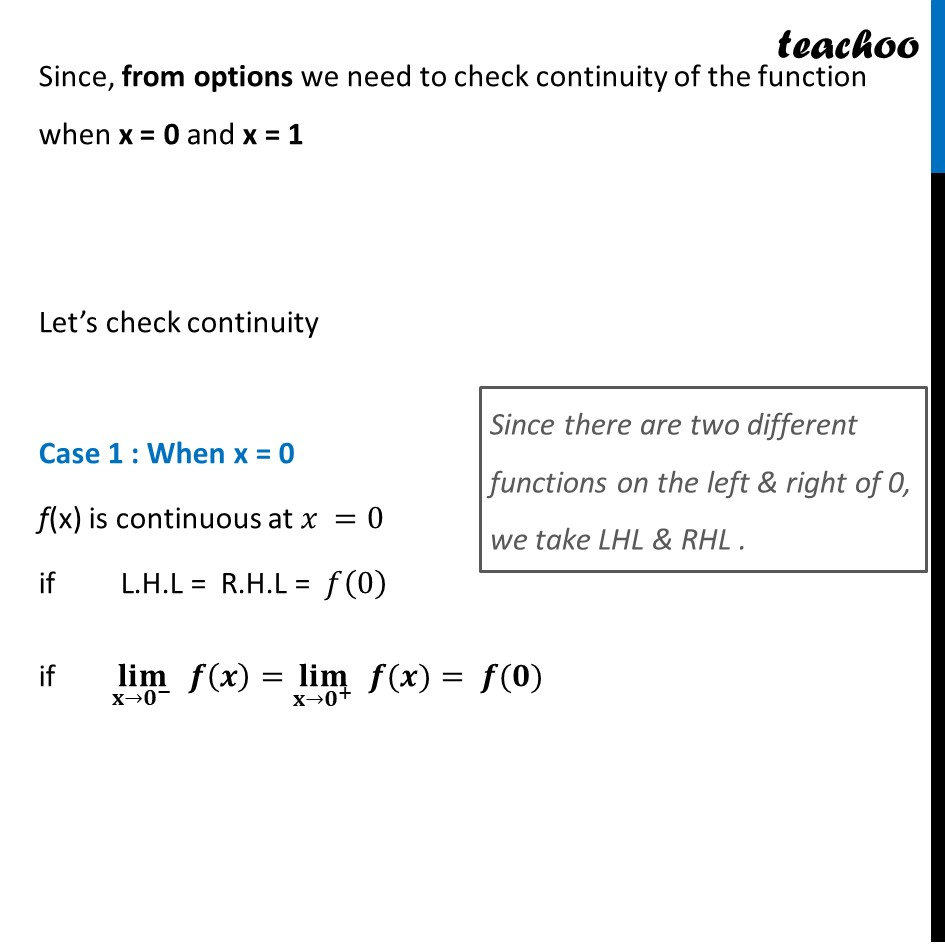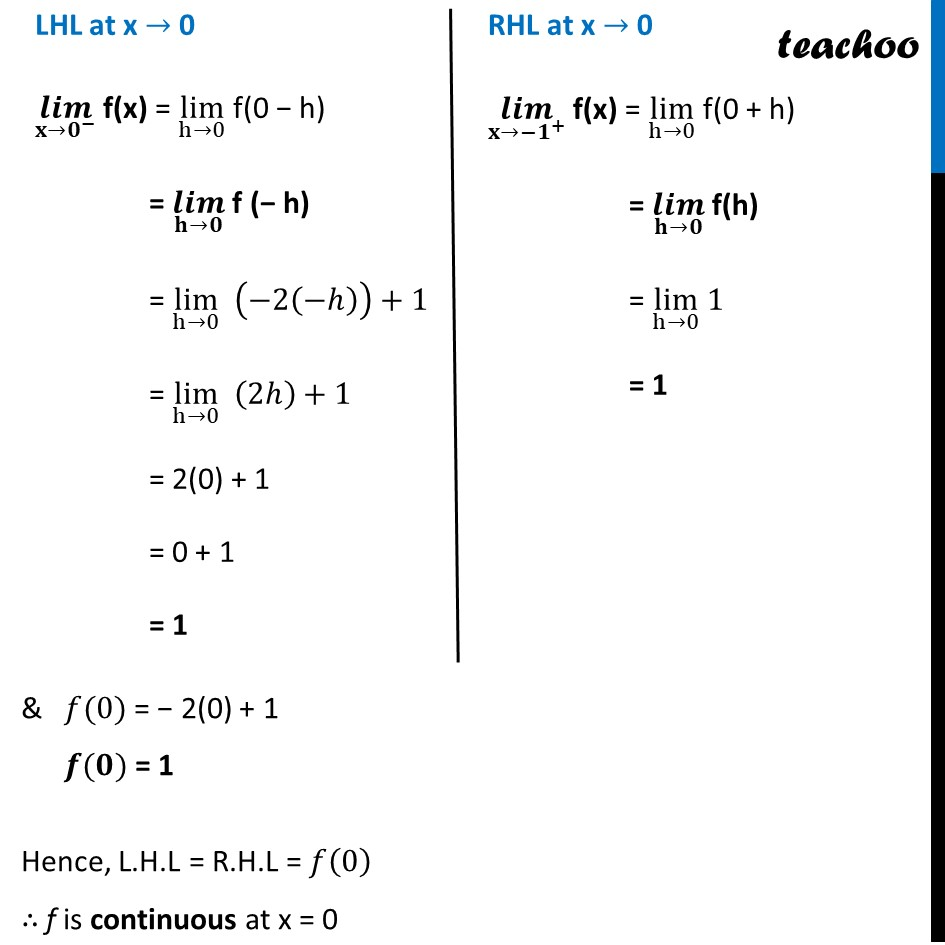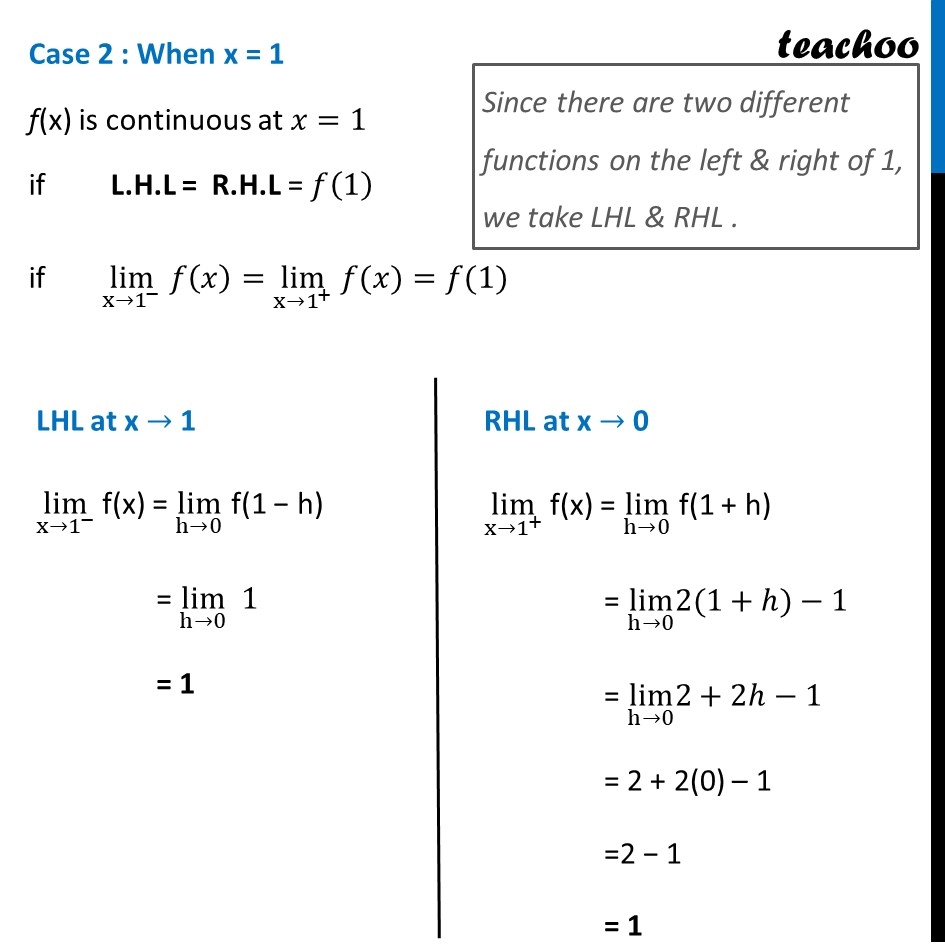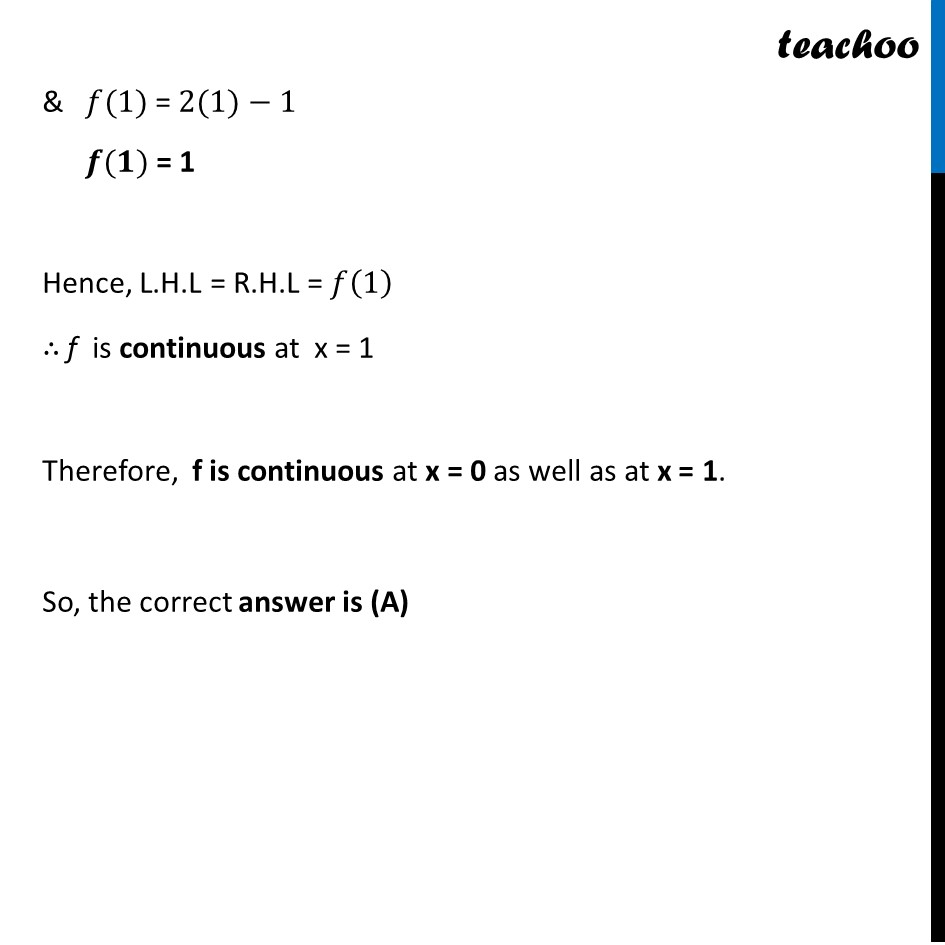Learn in your speed, with individual attention - Teachoo Maths 1-on-1 Class

### Transcript

Question 6 The function f (x) = |x| + |x – 1| is (A) continuous at x = 0 as well as at x = 1. (B) continuous at x = 1 but not at x = 0. (C) discontinuous at x = 0 as well as at x = 1. (D) continuous at x = 0 but not at x = 1. Given 𝑓(𝑥)= |𝑥|+|𝑥−1| Here, we have 2 critical points i.e. 𝑥 = 0, and 𝒙 = 1 So, our intervals will be When 𝒙≤𝟎 When 0<𝒙<𝟏 When 𝒙≥𝟏 When 𝒙≤𝟎 𝑓(𝑥)= |𝑥|+ |𝑥−1|. Here, both will be negative 𝑓(𝑥)=(−𝑥)+(−(𝑥−1)) 𝑓(𝑥)=−𝑥−(𝑥−1) 𝑓(𝑥)=−𝑥−𝑥+1 " " 𝒇(𝒙)=−𝟐𝒙+𝟏 When 0<𝒙<𝟏 𝑓(𝑥)= |𝑥| +|𝑥−1|. Here, x will be positive, but (x - 1) will be negative 𝑓(𝑥)=(𝑥)+(−(𝑥−1)) 𝑓(𝑥)=𝑥−(𝑥−1) 𝑓(𝑥)=𝑥−𝑥+1 " " 𝒇(𝒙)=𝟏 When 𝒙≥𝟏 𝑓(𝑥)= |𝑥|+|𝑥−1|. Here, both will be positive 𝑓(𝑥)=𝑥+(𝑥−1) 𝑓(𝑥)=𝑥+𝑥−1 " " 𝒇(𝒙)=𝟐𝒙−𝟏 Thus, our function becomes 𝒇(𝒙)={█(−𝟐𝒙+𝟏" " 𝒊𝒇 𝒙≤𝟎@ 𝟏 𝒊𝒇 𝟎<𝒙<𝟏@𝟐𝒙−𝟏 𝒊𝒇 𝒙≥𝟏)┤ Since, from options we need to check continuity of the function when x = 0 and x = 1 Let’s check continuity Case 1 : When x = 0 f(x) is continuous at 𝑥 =0 if L.H.L = R.H.L = 𝑓(0) if (𝐥𝐢𝐦)┬(𝐱→𝟎^− ) 𝒇(𝒙)=(𝐥𝐢𝐦)┬(𝐱→𝟎^+ ) " " 𝒇(𝒙)= 𝒇(𝟎) LHL at x → 0 (𝒍𝒊𝒎)┬(𝐱→𝟎^− ) f(x) = lim┬(h→0) f(0 − h) = (𝒍𝒊𝒎)┬(𝐡→𝟎) f (− h) = lim┬(h→0) (−2(−ℎ))+1 = lim┬(h→0) (2ℎ)+1 = 2(0) + 1 = 0 + 1 = 1 RHL at x → 0 (𝒍𝒊𝒎)┬(𝐱→〖−𝟏〗^+ ) f(x) = lim┬(h→0) f(0 + h) = (𝒍𝒊𝒎)┬(𝐡→𝟎) f(h) = lim┬(h→0) 1 = 1 Case 2 : When x = 1 f(x) is continuous at 𝑥=1 if L.H.L = R.H.L = 𝑓(1) if lim┬(x→1^− ) 𝑓(𝑥)=lim┬(x→1^+ ) " " 𝑓(𝑥)=𝑓(1) LHL at x → 1 lim┬(x→1^− ) f(x) = lim┬(h→0) f(1 − h) = lim┬(h→0) 1 = 1 RHL at x → 0 lim┬(x→1^+ ) f(x) = lim┬(h→0) f(1 + h) = lim┬(h→0) 2(1+ℎ)−1 = lim┬(h→0) 2+2ℎ−1 = 2 + 2(0) – 1 =2 − 1 = 1 & 𝑓(1) = 2(1)−1 𝒇(𝟏) = 1 Hence, L.H.L = R.H.L = 𝑓(1) ∴ f is continuous at x = 1 Therefore, f is continuous at x = 0 as well as at x = 1. So, the correct answer is (A)